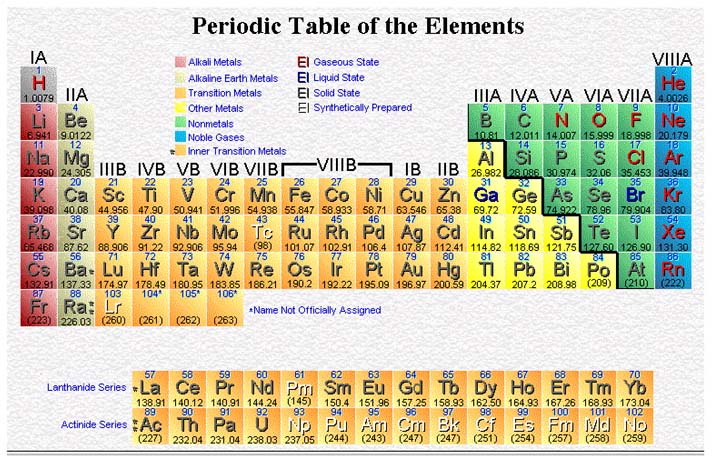CHEMISTRY Pre-AP Unit IV: Quantum Theory - Electronic Structure of the Atom Chapter 5Goal: The student will gain an understanding of the electronic structure of the atom.

Objectives: The student should be able to:

1. Describe what occurs as white light passes through a prism.

2. List in order, from shortest wavelength to longest wavelength, the types of radiation in the electromagnetic spectrum.

3. Relate the wavelength of a type of radiation to its energy and frequency.

4. Explain what happens when an atom absorbs light energy and name the state of the atom under these conditions.

5. Indicate why the spectrum of an element may be used to identify it.

6. In terms of the quantum theory, describe how atoms absorb and emit energy.

7. Define in relationship to Bohr’s model of the atom:
a) energy level b) ground state c) excited state

8. Explain how the photoelectric effect is induced by light energy.

9. Indicate what is meant by the duality of matter.

10. Discuss the wavelike nature of matter as proposed by De Broglie's Theory.

11. List one way that matter acts in a manner that reveals its wavelike nature.

12. State Heisenberg's Uncertainty Principle as it relates to atoms.

13. Indicate what information Schroedinger's Wave Equation gives us about the electron.

14. List the four quantum numbers and state what each number indicates about the electron.

15. Use the formula 2n2 to determine the maximum number of electrons for any energy level.

16. List the number of magnetic energy states in a sublevel.

17. List the four orbital types and indicate the shapes of their charge clouds and their values.

18. List the four orbital types in increasing order of energy.

19. State Pauli's Exclusion Principle and recognize the significance of this statement to electrons in the same atom.

20. Apply Hund's Rule to the filling of orbitals of an atom.

21. Using the Diagonal Rule, predict what orbitals will be filled first in an atom.

22. Give the electron configuration for elements when given the Atomic number (in both spectroscopic notation and pictorially).

23. List for any element the last energy level occupied by electrons and the number of electrons occupying the energy level.

24. Draw a dot diagram of an element to represent the valence electrons.

Activities:

Sep 26: Discuss Unit III Test, Begin Lecture Light and Quantized Energy

Sep 27: Finish Lecture Light and Quantized Energy, Begin Demonstration on Line Spectra

Sep 28: Finish Demonstration on Line Spectra, Mini-Lab: Flame Tests

Sep 29: Lecture Quantum Theory and the Atom

Oct 2: Lecture Aufbau Method and Orbital Diagrams

Oct 3: Lecture: Electron Configuration Notation, Electron-Dot Structures

Oct 4: Discuss homework and Review for Test

Oct 5: Unit IV Test

Homework:

Sep 26: Begin Chapter 5 Study Guide

Sep 27: Prelab for Flame Tests and Line Spectra

Sep 28: Work on Flame Tests Lab Report, Due on Oct 6

Sep 29: Work on Chapter 5 Study Guide

Oct 2: Finish lab report on Flame Tests and Line Spectra

Oct 3: Finish Chapter 5 Study Guide, Vocabulary and Concept Review

Oct 4: Study for test.